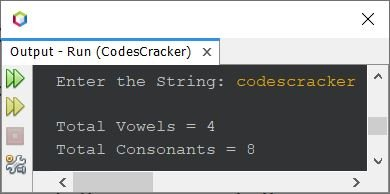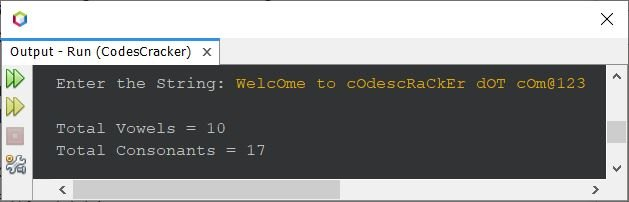# Java Program to Count Vowels and Consonants in a String

This article contains a program in Java that is used to count and print the total number of vowels and consonants available in a string. But before creating the actual program (the complete version), let's create a simple and basic version.

## Count Vowels and Consonants in String - Basic Version

The question is, write a Java program to count total vowels and consonants available in a string. The string must be received by user at run-time of the program. Here is its answer. I mean the program given below is its answer:

```import java.util.Scanner;

public class CodesCracker
{
public static void main(String[] args)
{
String str;
char ch;
int len, i, vowel=0, consonant=0;
Scanner s = new Scanner(System.in);

System.out.print("Enter the String: ");
str = s.nextLine();

len = str.length();
for(i=0; i<len; i++)
{
ch = str.charAt(i);
if(ch=='a'||ch=='e'||ch=='i'||ch=='o'||ch=='u')
vowel++;
else
consonant++;
}

System.out.println("\nTotal Vowels = " +vowel);
System.out.println("Total Consonants = " +consonant);
}
}```

Here is its sample run with user input codescracker:Now the problem is, what if user enters a string containing digits or special characters ?
also, what if user enters a string containing uppercase vowels ?
In those cases, above program will get failed to produce the correct output. Therefore let's modify the above program and create a new one.

## Count Vowels and Consonants in String - Complete Version

Since there are some limitations with above program. Therefore this program is created remove those limitations.

```import java.util.Scanner;

public class CodesCracker
{
public static void main(String[] args)
{
String str;
char ch;
int len, i, vowel=0, consonant=0;
Scanner s = new Scanner(System.in);

System.out.print("Enter the String: ");
str = s.nextLine();

len = str.length();
for(i=0; i<len; i++)
{
ch = str.charAt(i);
if(ch=='a'||ch=='e'||ch=='i'||ch=='o'||ch=='u')
vowel++;
else if(ch=='A'||ch=='E'||ch=='I'||ch=='O'||ch=='U')
vowel++;
else
{
int ascii = ch;
if((ascii>=65 && ascii<=90) || (ascii>=97 && ascii<=122))
consonant++;
}
}

System.out.println("\nTotal Vowels = " +vowel);
System.out.println("Total Consonants = " +consonant);
}
}```

Here is its sample run with user input WelcOme to cOdescRaCkEr dOT cOm@123 as string to count total number of vowels and consonants available in it:In the string WelcOme to cOdescRaCkEr dOT cOm@123, alphabets are WelcOmetocOdescRaCkErdOTcOm. In which, vowels are eOeoOeaEOo and consonants are WlcmtcdscRCkrdTcm. That is, 10 vowels and 17 consonants.

In above program, I've used ASCII value of alphabets to match. The ASCII values of A-Z are 65-90, where ASCII values of a-z are 97-122.

From above program, the following set of statements:

```if(ch=='a'||ch=='e'||ch=='i'||ch=='o'||ch=='u')
vowel++;
else if(ch=='A'||ch=='E'||ch=='I'||ch=='O'||ch=='U')
vowel++;```

can be replaced with the set of statements given below:

```if(ch=='a'||ch=='e'||ch=='i'||ch=='o'||ch=='u'||ch=='A'||ch=='E'||ch=='I'||ch=='O'||ch=='U')
vowel++;```

I've not chosen this to avoid lengthy single line code. But you can, if you want.

Java Online Test

« Previous Program Next Program »